# Taylor series operator commutes with differentiation

## Statement

Suppose$f$ if a function defined on a subset of the reals that is infinitely differentiable at a point$x_0$ in its domain. Then, the derivative$f'$ is also defined and infinitely differentiable at$x_0$, and the Taylor series for$f'$ is the derivative (in the sense of derivative of power series) of the Taylor series for$f$.

## Proof

Given:$f$ is a function defined on a subset of the reals and it is infinitely differentiable at a point$x_0$ in its domain.

To prove: The derivative$f'$ is defined and infinitely differentiable at$x_0$ and the Taylor series for$f'$ is the derivative of the Taylor series for$f$.

Proof: We have the following relation between the derivatives of$f$ and$f'$:$(f')^{(k)}(x_0) = f^{(k+1)}(x_0) \ \forall \ k \in \{ 0,1,2,\dots,\} \qquad (\dagger)$

In particular, this means that, since$f$ is infinitely differentiable at$x_0$, so is$f'$, with the derivatives given by the above relationship. Thus, it makes sense to take the Taylor series of$f'$.

### Derivative of the Taylor series

We note that: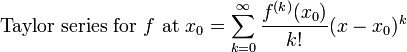$\mbox{Taylor series for } f \mbox{ at } x_0 = \sum_{k=0}^\infty \frac{f^{(k)}(x_0)}{k!}(x - x_0)^k$

Let's try differentiating this. Differentiation of power series is term-wise, so we compute the derivative for each term, i.e., we try to calculate:$\frac{d}{dx}\left[\frac{f^{(k)}(x_0)}{k!}(x - x_0)^k\right]$

The expression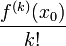$\frac{f^{(k)}(x_0)}{k!}$ is constant, so we use the differentiation rule for power functions on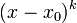$(x - x_0)^k$ (with the chain rule) and obtain: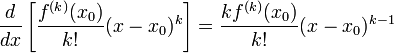$\frac{d}{dx}\left[\frac{f^{(k)}(x_0)}{k!}(x - x_0)^k\right] = \frac{kf^{(k)}(x_0)}{k!}(x - x_0)^{k-1}$

For$k = 0$, this becomes 0. For$k \ge 1$, set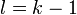$l= k - 1$ (note that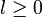$l \ge 0$ now) and this becomes:$\frac{f^{(l + 1)}(x_0)}{l!}(x - x_0)^l$

Now adding together all the terms, we get: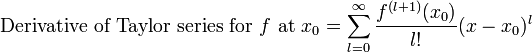$\mbox{Derivative of Taylor series for } f \mbox{ at } x_0 = \sum_{l=0}^\infty \frac{f^{(l + 1)}(x_0)}{l!}(x - x_0)^l$

Since$l$ is a dummy variable, we can use the dummy variable$k$ instead, and get:$\mbox{Derivative of Taylor series for } f \mbox{ at } x_0 = \sum_{k=0}^\infty \frac{f^{(k + 1)}(x_0)}{k!}(x - x_0)^k$

### Taylor series of the derivative

We note that:$\mbox{Taylor series for } f' \mbox{ at } x_0 = \sum_{k=0}^\infty \frac{(f')^{(k)}(x_0)}{k!}(x - x_0)^k$

By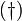$(\dagger)$, we can rewrite$(f')^{(k)}(x_0)$ on the right side as$f^{(k+1)}(x_0)$, and get: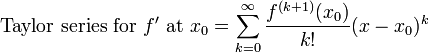$\mbox{Taylor series for } f' \mbox{ at } x_0 = \sum_{k=0}^\infty \frac{f^{(k+1)}(x_0)}{k!}(x - x_0)^k$

### Checking equality

It is now easy to verify that we have precisely the same expressions for the derivative of the Taylor series of$f$ and the Taylor series of$f'$.